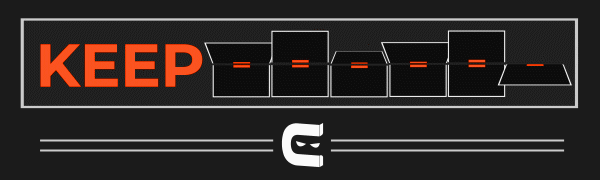New update is available. Click here to update.

# Factorial of a Number

## Introduction

In this blog, we will discuss the problem of calculating the factorial of a number. We will code the solution to this problem in C language. We will discuss two different approaches to solve this problem. But before discussing the different solutions to this problem, we should first look at a factorial of a number and how we can calculate the factorial of a number.

Also see, Binary to Hex Converter.

What is Factorial of a number?

Factorial of a number can be defined as the product of all the numbers less than or equal to the given number. If there is a number n, its factorial can be represented as n!. For example, if we need to calculate the factorial of the number 5, we will represent the factorial of 5 as 5!. There is a universal fact that

Now, let us look at the formula with which we can calculate the factorial of a number,

### Sample Examples

Example 1:

``````Input:
N = 6

Output:
720

Explanation:
6! = 6 x 5 x 4 x 3 x 2 x 1 = 720``````

Example 2:

``````Input:
N = 8

Output:
40320

Explanation:
8! = 8 x 7 x 6 x 5 x 4 x 3 x 2 x 1 = 40320``````

## Iterative Method

1. In this approach, we will calculate the factorial of a number using a for a loop. First, we will declare a variable named and a variable named fact which will be of long data type.
2. Let us suppose that value of n is equal to 4. Then, we will start the for loop from 1 till 4, and we will keep multiplying the numbers. Simultaneously we will store the result in the variable fact.
3. We will print the result variable when the loop ends, i.e., fact.

### Implementation in C++

``````// C program to calculate the factorial of a number
#include <stdio.h>

int main()
{
long int n, fact = 1;

scanf("%d", &n);

for (int i = 1; i <= n; i++)
{
fact = fact * i;
}
printf("Factorial of %d is equal to %d", n, fact);

return 0;
}``````

Output:

``````Input: N = 7

Output: Factorial of 7 is equal to 5040 ``````

#### Complexity Analysis

Time Complexity: O(N)

Since we are traversing a for loop from 1 to n, the complexity would be O(N).

Space Complexity: O(1)

## Factorial of Number using Recursion

1. In this approach, we will calculate the factorial of a number using Recursion. First, we will write the base condition for recursive function, i.e., if n is equal to 0, then return 1.
2. After that, we will make the recursive call in which the function will call itself by decreasing the value of n by 1. It would look like this n*factorial(n-1)  here factorial is the function where the recursive call is being made.
3. Now in the main function, we will declare a variable named fact, and we will assign the returned value of this function factorial.

Below is the illustration of how the function will calculate the factorial of 5

### Implementation in C++

``````// C program to calculate the factorial of a number
#include <stdio.h>

// function to make recursive calls
long factorial(long int n){

//base case
if(n==0){
return 1;
}

// recursive call to calculate the factorial
return n*factorial(n-1);
}
int main()
{
long int n, fact;

scanf("%d", &n);

// storing the result from the function in the variable
fact = factorial(n);

// printing the result
printf("Factorial of %d is equal to %d", n, fact);

return 0;
}``````

Output:

``````Input: N=3
Output: Factorial of 3 is equal to 6 ``````

#### Complexity Analysis

Time Complexity: O(N)

Since we are making recursive calls equal to N, we can say that the time complexity would be equal to O(N).

Space Complexity: O(1)

## FAQs

1. What do you mean by the scope of the variable? What is the scope of the variable in C?
The variable's scope may be defined as the part of the code area where the variables specified in the program can be directly accessed. Every identifier in C is lexically (or statically) scoped.

2. Why is C called the mother of all Languages?
C introduced several fundamental concepts and data structures like arrays, lists, functions, strings, etc. Many languages developed after C are based on the C language. As a result, it is considered the mother of all languages.

3. What is the use of functions in C?
→ C functions are utilized in our application to repeatedly prevent rewriting the same code.
→ C functions can be called as many times as we want from wherever our application is.
→ When we break a program into functions, we can track any portion of our program.
→ C functions give the idea of reusability, i.e., they divide extensive work into smaller ones, making the C program more intelligible.

## Key takeaways

This article discussed the problem of calculating the factorial of a number. We have discussed two approaches to this problem: the first approach using a loop and the second approach using Recursion. We have also discussed the time complexities of all the approaches.

Also check out - Rod Cutting Problem

I hope you must have gained some knowledge after reading this problem, and now it is your turn to code this problem in your way

Until then, Keep Learning and Keep Coding.